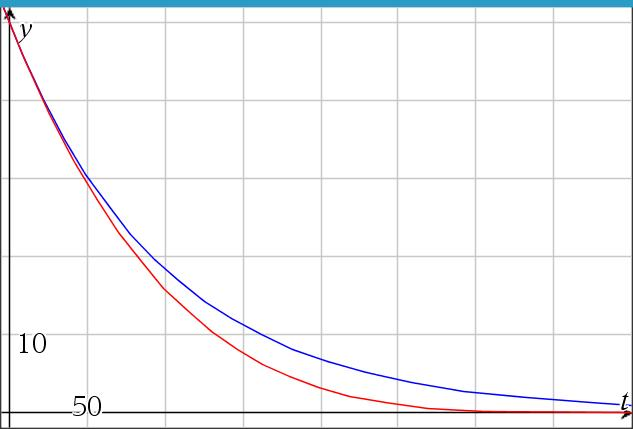# Brine mixture, case 2, inflow≠outflow, pure water in

Up a level : Differential Equations
Previous page : Brine mixture, case 1, inflow=outflow, pure water in
Next page : The limit of case 2Case 2: r1r2, k1=0

Here the rate of the inflow and the outflow differs. The volume will thus be$V = {V_0} - \left( {{r_2} - {r_1}} \right)t$

This will give us$\frac{{dy}}{{dt}} = - \frac{y}{V}{r_2} = - \frac{y}{{{V_0} - \left( {{r_2} - {r_1}} \right)t}}{r_2}$

This is also a separable equation. We get$\frac{{dy}}{y} = - \frac{{{r_2}}}{{{V_0} - \left( {{r_2} - {r_1}} \right)t}}dt$

Integrating both sides will give us$\ln y = \frac{{{r_2}}}{{{r_2} - {r_1}}}\ln \left( {{V_0} - \left( {{r_2} - {r_1}} \right)t} \right) + {C_1}$

(Yet agin we don’t need to care about the absolute sign since we cannot have negative volumes.)

This gives us$\ln y = \ln {\left( {{V_0} - \left( {{r_2} - {r_1}} \right)t} \right)^{\frac{{{r_2}}}{{{r_2} - {r_1}}}}} + {C_1}$

or$y = {e^{{C_1}}}{\left( {{V_0} - \left( {{r_2} - {r_1}} \right)t} \right)^{\frac{{{r_2}}}{{{r_2} - {r_1}}}}} = C{\left( {{V_0} - \left( {{r_2} - {r_1}} \right)t} \right)^{\frac{{{r_2}}}{{{r_2} - {r_1}}}}}$

This might look fairly messy, but as we can see, given reasonable numbers, it will turn out rather OK.

An example

Say we have V0=2000 l, r1 =15 l/s, r2 = 20 l/s, k1=0 kg/l and y0 = 50 kg. This would give us the solution$y = {C_2}{\left( {2000 - \left( {20 - 15} \right)t} \right)^{\frac{{20}}{{20 - 15}}}} = {C_2}{\left( {2000 - 5t} \right)^4}$

Finally, we need to find C, but to make that somewhat easier we can first move out a factor of 20004 out of the bracket.${y_0} = 50 = {C_2} \cdot {2000^4}{\left( {1 - \frac{0}{{400}}} \right)^4}$

We can then let the constant C2 absorb the 20004 to get us$y = C \cdot {\left( {1 - \frac{5}{{2000}}t} \right)^4} = {y_0}{\left( {1 - \frac{t}{{400}}} \right)^4} = 50{\left( {1 - \frac{t}{{400}}} \right)^4}$

Let us compare this to example solution for case 1. The red curve is our case 2.We can here see that the solutions are quite similar, but that the amount of salt goes to 0 more quickly. After 2000/5=400 second the volume is 0, and we could thus not have any salt left.

Back to the general solution

We can go back to our general solution to get$\begin{array}{l}y = {C_2}{\left( {{V_0} - \left( {{r_2} - {r_1}} \right)t} \right)^{\frac{{{r_2}}}{{{r_2} - {r_1}}}}} = {C_2}V_0^4{\left( {1 - \frac{{{r_2} - {r_1}}}{{{V_0}}}t} \right)^{\frac{{{r_2}}}{{{r_2} - {r_1}}}}}\\ \;\;\; = {y_0}{\left( {1 - \frac{{{r_2} - {r_1}}}{{{V_0}}}t} \right)^{\frac{{{r_2}}}{{{r_2} - {r_1}}}}} \end{array}$Up a level : Differential Equations
Previous page : Brine mixture, case 1, inflow=outflow, pure water in
Next page : The limit of case 2Last modified: Dec 28, 2020 @ 11:14